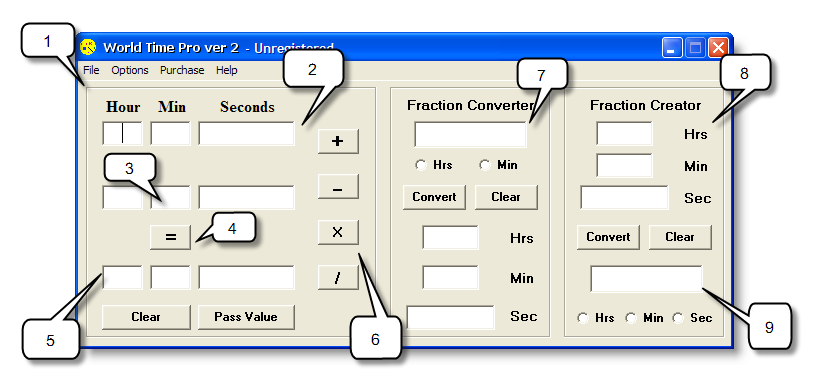# The World Time Pro window

This topic describes the buttons and fields in the World Time Pro window.

## The World Time Pro windowField or button Number Function
Main calculation pane 1 Contains the buttons and fields to perform addition, subtraction, multiplication and division of units of time.
Upper row of entry fields 2 Specifies the first period of time in the calculation.
Lower row of entry fields 3 Specifies the second period of time in the calculation.
Note: If you click the Multiply or Divide buttons, the lower row of entry fields are replaced by the Constant field.
Equals button 4 Performs the calculation.
Result fields 5 Display the result of the calculation.
Operation buttons 6 Specifies if the calculation is addition (+), subtraction (-), multiplication (x) or division (/).
Clear button n/a Deletes all values from the pane.
Pass Value button n/a Moves the value in the result fields to the upper row of entry fields.
Fraction Converter pane 7 Contains the buttons and fields to convert fractions into periods of time.
Fraction Converter field n/a Specifies the fraction to be converted into a period of time.
Note: You specify if the fraction is in hours or minutes by selecting either Hrs or Min below this field.
Convert button n/a In the Fraction Converter pane, converts a fraction into a period of time. In the Fraction Creator pane, converts a period of time into a fraction.
Fraction Creator pane 8 Contains the buttons and fields to convert periods of time into fractions.
Fraction Creator result field 9 Displays the period of time as a fraction of hours, minutes or seconds.
Note: You specify if the fraction is in hours, minutes or seconds by selecting either Hrs, Min or Sec below this field.